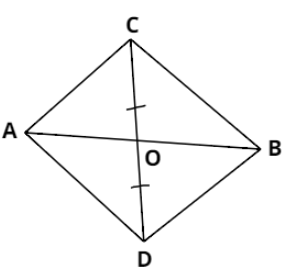Courses
Courses for Kids
Free study material
Offline Centres
MoreLast updated date: 01st Dec 2023
Total views: 382.8k
Views today: 11.82k

# In the given figure, ABC and ABD are two triangles on the same base AB. If the line-segment CD is bisected by AB at O, show that area of ($\Delta ABC$) $=$ area of ($\Delta ABD$).Verified
382.8k+ views
Hint: Since line AB is bisecting line CD, O is the midpoint of CD. Therefore AO and OB are the medians of triangles ACD and BCD. Use the property that a median divides a triangle into two parts of equal areas.

According to the question, line segment CD is bisected by AB at point O. So, we have:
$\Rightarrow OC = OD$
In $\Delta ACD$, we have $OC = OD$. Therefore AO is the median of $\Delta ACD$.
We know that a median divides a triangle into two triangles of equal areas. So we have:
$\therefore {\text{ar}}\left( {\Delta AOC} \right) = {\text{ar}}\left( {\Delta AOD} \right) .....(i)$
Similarly in $\Delta BCD$, $BO$ is the median because CD is bisected by AB at O. So, we have:
$\therefore {\text{ar}}\left( {\Delta BOC} \right) = {\text{ar}}\left( {\Delta BOD} \right) .....(i)$
Adding equation $(i)$ and $(ii)$, we’ll get:
$\Rightarrow {\text{ar}}\left( {\Delta AOC} \right) + {\text{ar}}\left( {\Delta BOC} \right) = {\text{ar}}\left( {\Delta AOD} \right) + {\text{ar}}\left( {\Delta BOD} \right) .....(iii)$
And from the figure we can say that ${\text{ar}}\left( {\Delta AOC} \right) + {\text{ar}}\left( {\Delta BOC} \right) = ar\left( {\Delta ABC} \right)$ and ${\text{ar}}\left( {\Delta AOD} \right) + {\text{ar}}\left( {\Delta BOD} \right) = ar\left( {\Delta ABD} \right)$. So putting these values in equation $(iii)$, we’ll get:
$\Rightarrow ar\left( {\Delta ABC} \right) = ar\left( {\Delta ABD} \right)$.
This is the required proof.

Note: A median of a triangle is a line drawn from a vertex of the triangle to the third side dividing it into two equal parts. Thus, a triangle can have three different medians. Medians also divide the triangle into two parts of equal area.